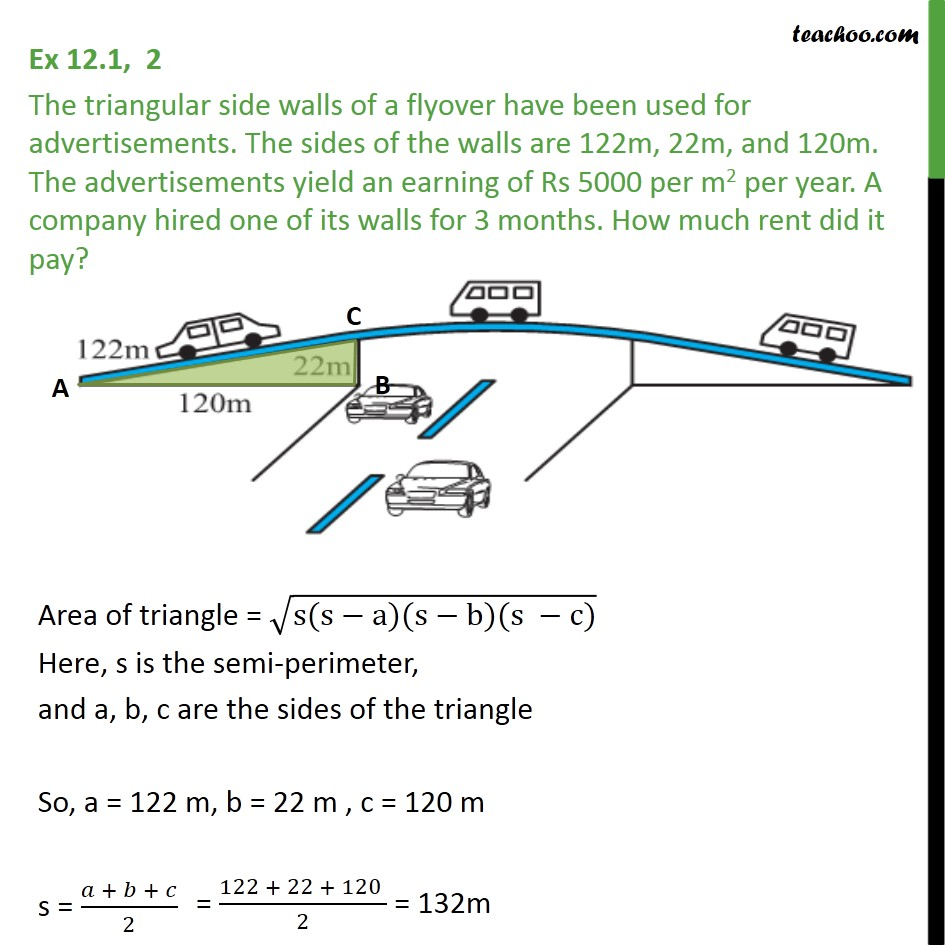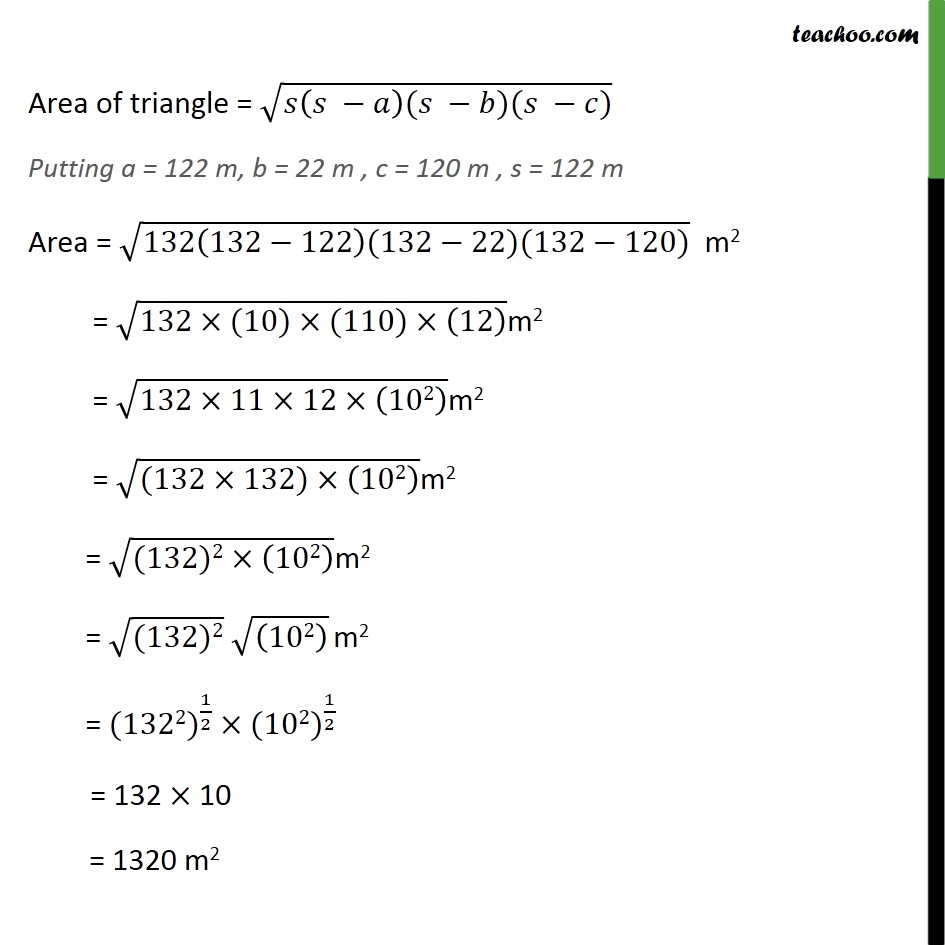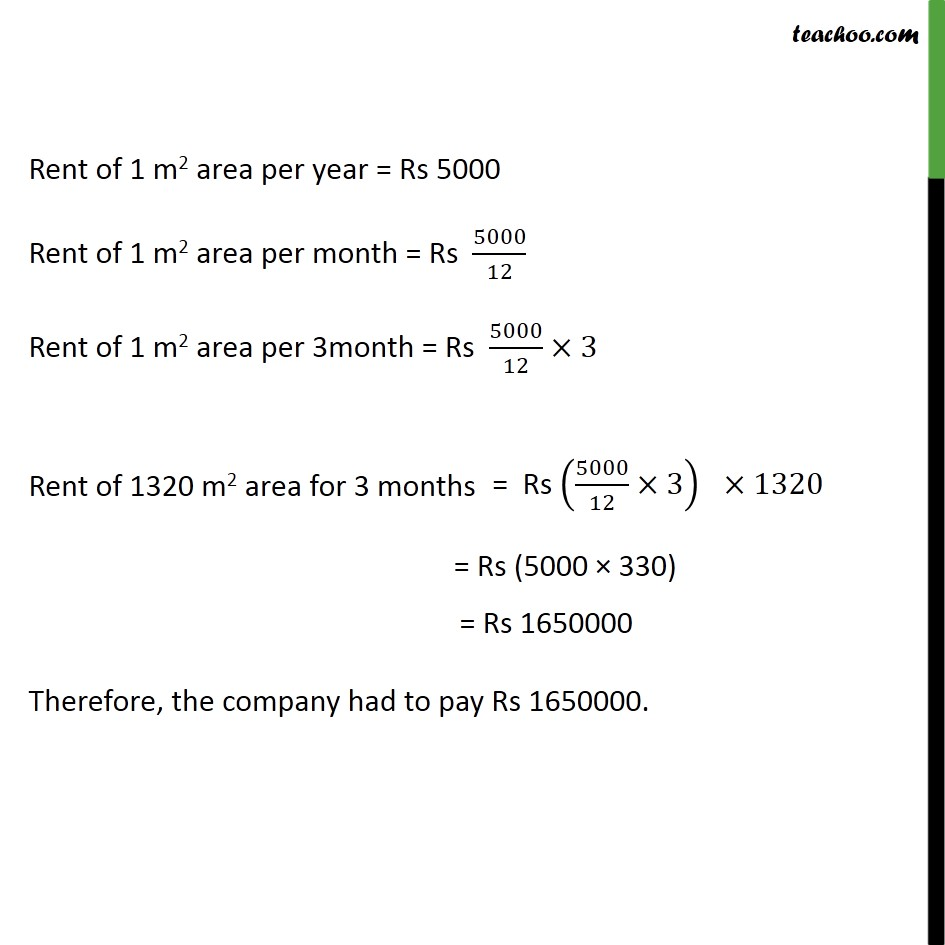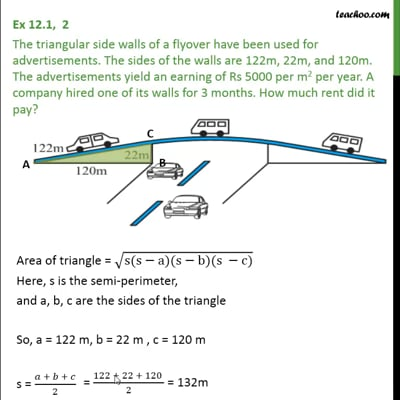Ex 12.1

Chapter 12 Class 9 Herons Formula
Serial order wiseThis video is only available for Teachoo black users

Solve all your doubts with Teachoo Black (new monthly pack available now!)

### Transcript

Ex 12.1, 2 The triangular side walls of a flyover have been used for advertisements. The sides of the walls are 122m, 22m, and 120m. The advertisements yield an earning of Rs 5000 per m2 per year. A company hired one of its walls for 3 months. How much rent did it pay? Area of triangle = √(𝑠(𝑠 −𝑎)(𝑠 −𝑏)(𝑠 −𝑐)) Putting a = 122 m, b = 22 m , c = 120 m , s = 122 m Area = √(132(132−122)(132−22)(132−120)) m2 = √(132×(10)×(110)×(12) )m2 = √(132×11×12×(102) )m2 = √((132×132)×(102) )m2 = √((132)2×(102) )m2 = √((132)2) √((102) ) m2 = 〖(1322)〗^(1/2) × 〖(102)〗^(1/2) = 132 × 10 Rent of 1 m2 area per year = Rs 5000 Rent of 1 m2 area per month = Rs 5000/12 Rent of 1 m2 area per 3month = Rs 5000/12 ×3 Rent of 1320 m2 area for 3 months = Rs (5000 × 330) Therefore, the company had to pay Rs 1650000.